# What Are The Output Values Of Sine? (5 Key Values To Know)

In trigonometry, we often use common input angles (such as 30 or 60 degrees) for the sine function.  It helps to know the corresponding outputs for these common angles to increase your calculation speed.

So, what are the output values of sine?  Five output values of sine you should know are sin(0) = 0, sin(π/6) = 1/2, sin(π/4) = √2/2, sin(π/3) = √3/2, and sin(π/2) = 1. These output values correspond to the common degree measures 0o, 30o, 45o, 60o, and 90o. Using the negative of these angles as input gives us the opposite (negative) outputs.

Of course, when the input angle for sine is in quadrants 1 or 2 (angles between 0o and 180o, or 0 to π radians), we get positive values for sine.  When the input angle for sine is in quadrants 3 or 4 (angles between 180o and 360o, or π to 2π radians), we get negative values for sine.

In this article, we’ll talk about output values for sine and how to remember the 5 common ones mentioned above.  We’ll also review the signs of the outputs and when they are positive, negative, or zero.

Let’s get started.

## What Are The Output Values Of Sine?

The output values of sine are given in the table below for 5 common values:

As an easy way to remember the values, note that each output value has the form √n/2, where n goes from 0 to 4 as we move from the degree measures 0 to 90o (0 to π/2 radians):

• For 0o: use n = 0, so the output is sin(0o) = √0/2 = 0/2 = 0.
• For 30o: use n = 1, so the output is sin(30o) = √1/2 = 1/2.
• For 45o: use n = 2, so the output is sin(45o) = √2/2.
• For 60o: use n = 3, so the output is sin(60o) = √3/2.
• For 90o: use n = 4, so the output is sin(90o) = √4/2 = 2/2 = 1.

Remember that we measure reference angles from the x-axis to get an acute angle (less than 90 degrees).  This means that in the 2nd quadrant, we subtract the angle from 180 degrees (π radians) to get our reference angle.

Trigonometric Function in S7 1200 P...
Trigonometric Function in S7 1200 PLC - SIN, COS, TAN, ASIN, ACOS, ATAN

Also, keep in mind that the output values of sine are positive in the 2nd quadrant.

So, in the 2nd quadrant, we would get the following reference angles and output values for sine:

In the 3rd quadrant, we subtract 180 degrees (π radians) from the angle to get our reference angle.

In the 3rd quadrant, we would get the following reference angles and output values for sine:

In the 4th quadrant, we subtract the angle from 360 degrees (2π radians) to get our reference angle.

Also, the output values of sine are negative in the 4th quadrant.

In the 4th quadrant, we would get the following reference angles and output values for sine:

Finally, remember that for a negative angle, we add 360 degrees (2π radians) until we get to an angle between 0o and 360o.

For an angle greater than 360o, we do the opposite and subtract 360 degrees (2π radians) until we get to an angle between 0o and 360o.

### Examples Of Output Values Of Sine

Let’s take a look at some examples to show how to find the output values of sine for various input angles.

#### Example 1: Sine In The First Quadrant

Let’s say that we have the angle A = 60o, which is in the first quadrant (it is between 0o and 90o).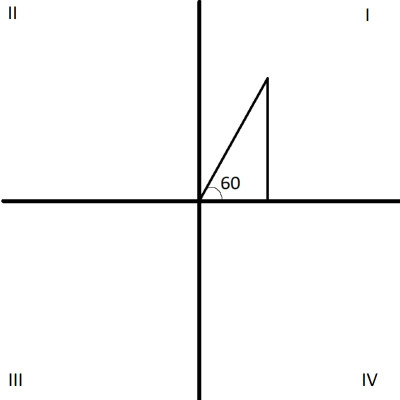A 60 degree angle is in the first quadrant (quadrant I). We can look up the value of sine for 60 degrees in a table.

To find the sine of the angle A, all we need to do is look up the value in the first table above.

So:

• sin(A)
• =sin(60o)
• =√3/2

#### Example 2: Sine In The Second Quadrant

Let’s say that we have the angle A = 150o, which is in the second quadrant (it is between 90o and 180o).

To find the sine of the angle A, we will first need to find the reference angle A’.  In the second quadrant, we calculate

• A’ = 180o – A
• A’ = 180o – 150o
• A’ = 30o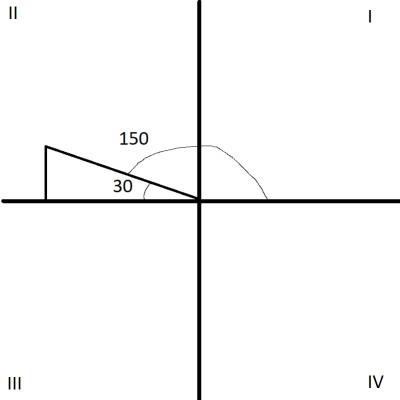A 150 degree angle is in the second quadrant (quadrant II). We can look up the value of the reference angle (30 degrees) in a sine table.

Also, we know that sine has positive output in the 2nd quadrant.  Now, we need to look up the value in the first table above.

So:

• sin(A)
• =sin(150o)
• =sin(30o)  [using our reference angle, A’ = 30o, and sine is positive in the 2nd quadrant]
• =1/2  [sin(30o) = 1/2, from the table above]

#### Example 3: Sine In The Third Quadrant

Let’s say that we have the angle A = 225o, which is in the third quadrant (it is between 180o and 270o).

To find the sine of the angle A, we will first need to find the reference angle A’.  In the third quadrant, we calculate

• A’ = A – 180o
• A’ = 225o – 180o
• A’ = 45o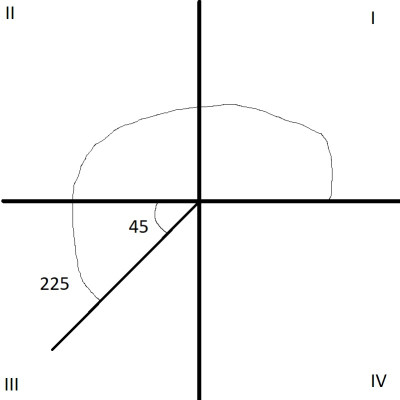A 225 degree angle is in the third quadrant (quadrant III). We can look up the value of the reference angle (45 degrees) in a sine table.

Also, we know that sine has negative output in the 3rd quadrant.  Now, we need to look up the value in the first table above.

So:

• sin(A)
• =sin(225o)
• =-sin(45o)  [using our reference angle, A’ = 45o, and sine is negative in the 3rd quadrant]
• =-√2/2  [sin(45o) = √2/2, from the table above]

#### Example 4: Sine In The Fourth Quadrant

Let’s say that we have the angle A = 330o, which is in the fourth quadrant (it is between 270o and 360o).

To find the sine of the angle A, we will first need to find the reference angle A’.  In the fourth quadrant, we calculate

• A’ = 180o – A
• A’ = 360o – 330o
• A’ = 30o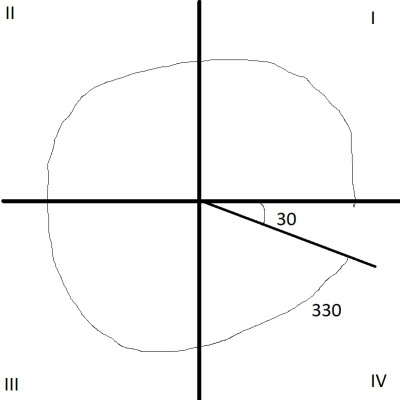A 330 degree angle is in the fourth quadrant (quadrant IV). We can look up the value of the reference angle (30 degrees) in a sine table.

Also, we know that sine has negative output in the 4th quadrant.  Now, we need to look up the value in the first table above.

So:

• sin(A)
• =sin(330o)
• =-sin(30o)  [using our reference angle, A’ = 30o, and sine is negative in the 4th quadrant]
• =-1/2  [sin(30o) = 1/2, from the table above]

#### Example 5: Sine Of A Negative Angle

Let’s say that we have the angle A = -150o, which is negative.  So, we will add 360o until we get an angle measure between 0o and 360o.

This gives us B = -150o + 360o = 210o.

To find the sine of the angle A = -150o, we can find the sine of angle B = 210o (note that B = 210o is in the 3rd quadrant, since it is between 180o and 270o).

First, we need to find the reference angle B’.  In the 3rd quadrant, we calculate

• B’ = B – 180o
• B’ = 210o – 180o
• B’ = 30o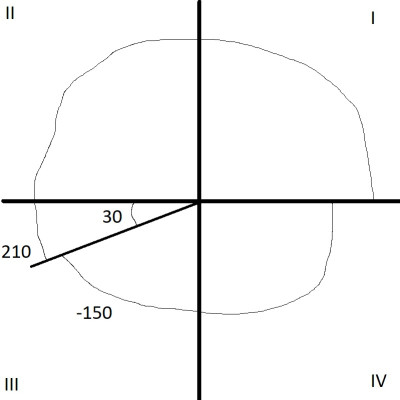A -150 degree angle is in the third quadrant (quadrant III), and is equivalent to an angle of 210 degrees. We can look up the value of the reference angle (30 degrees) in a sine table.

Also, we know that sine has negative output in the 3rd quadrant.  Now, we need to look up the value in the first table above.

So:

• sin(A)
• =sin(B)
• =sin(210o)
• =-sin(30o)  [using our reference angle, B’ = 30o, and sine is negative in the 3rd quadrant]
• =-1/2  [sin(30o) = 1/2, from the table above]

#### Example 6: Sine Of An Angle Greater Than 360o

Let’s say that we have the angle A = 630o, which is greater than 360o.  So, we will subtract 360o until we get an angle measure between 0o and 360o.

This gives us B = 630o – 360o = 270o.

To find the sine of the angle A = 630o, we can find the sine of angle B = 270o (note that B = 270o is on the border between the 3rd and 4th quadrants, since 270o is the cutoff).

First, we need to find the reference angle B’.  We calculate

• B’ = B – 180o
• B’ = 270o – 180o
• B’ = 90o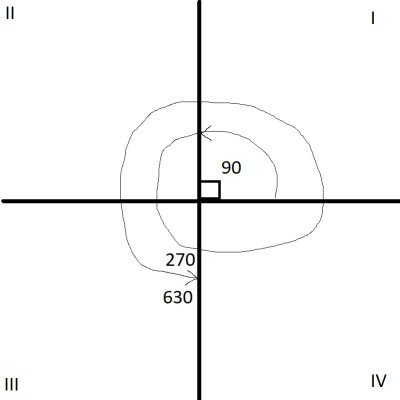A 630 degree angle is in the third quadrant (quadrant III), and is equivalent to an angle of 270 degrees. We can look up the value of the reference angle (90 degrees) in a sine table.

Also, we know that sine has negative output in the 3rd quadrant.  Now, we need to look up the value in the first table above.

So:

• sin(A)
• =sin(B)
• =sin(270o)
• =-sin(90o)  [using our reference angle, B’ = 90o, and sine is negative in the 3rd quadrant]
• =-1  [sin(90o) = 1, from the table above]

## Conclusion

Now you know more about the output values of sine and how they change as input angles change.  You also know how to figure out the sign of the outputs, based on the quadrant of the input angle.

You can learn about the output values of cosine here.

You can learn more about uses of trig functions here.

I hope you found this article helpful.  If so, please share it with someone who can use the information.